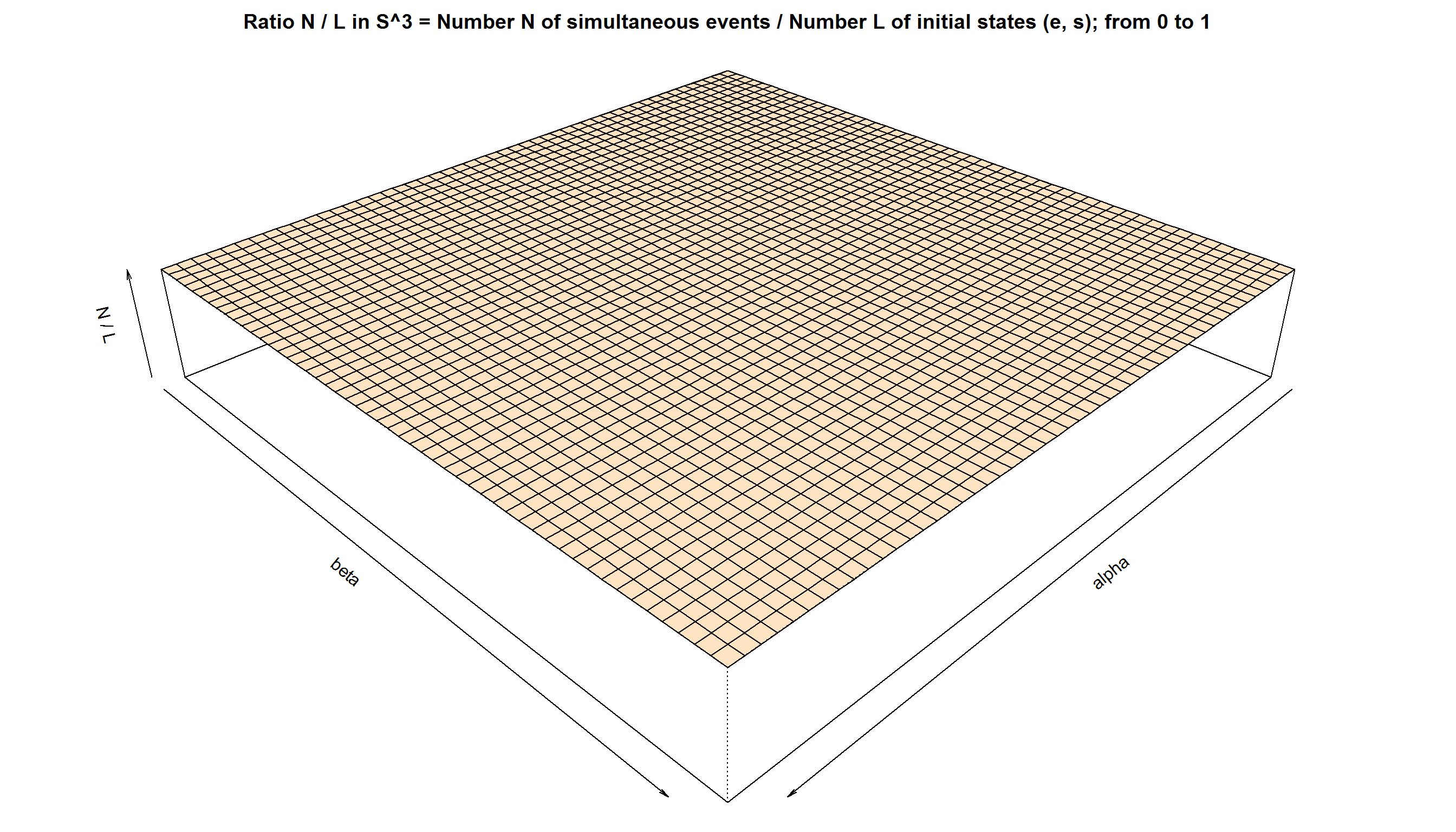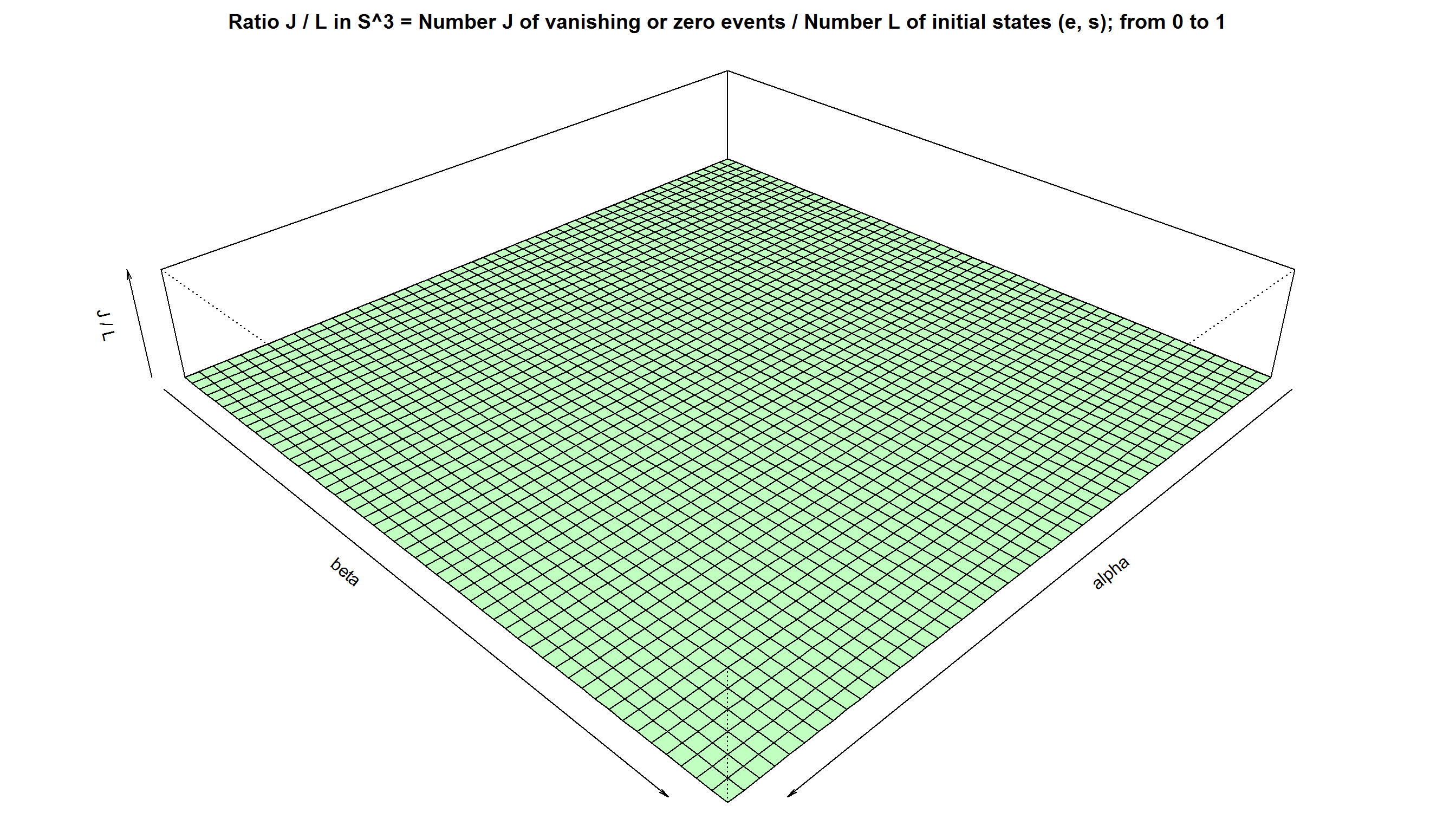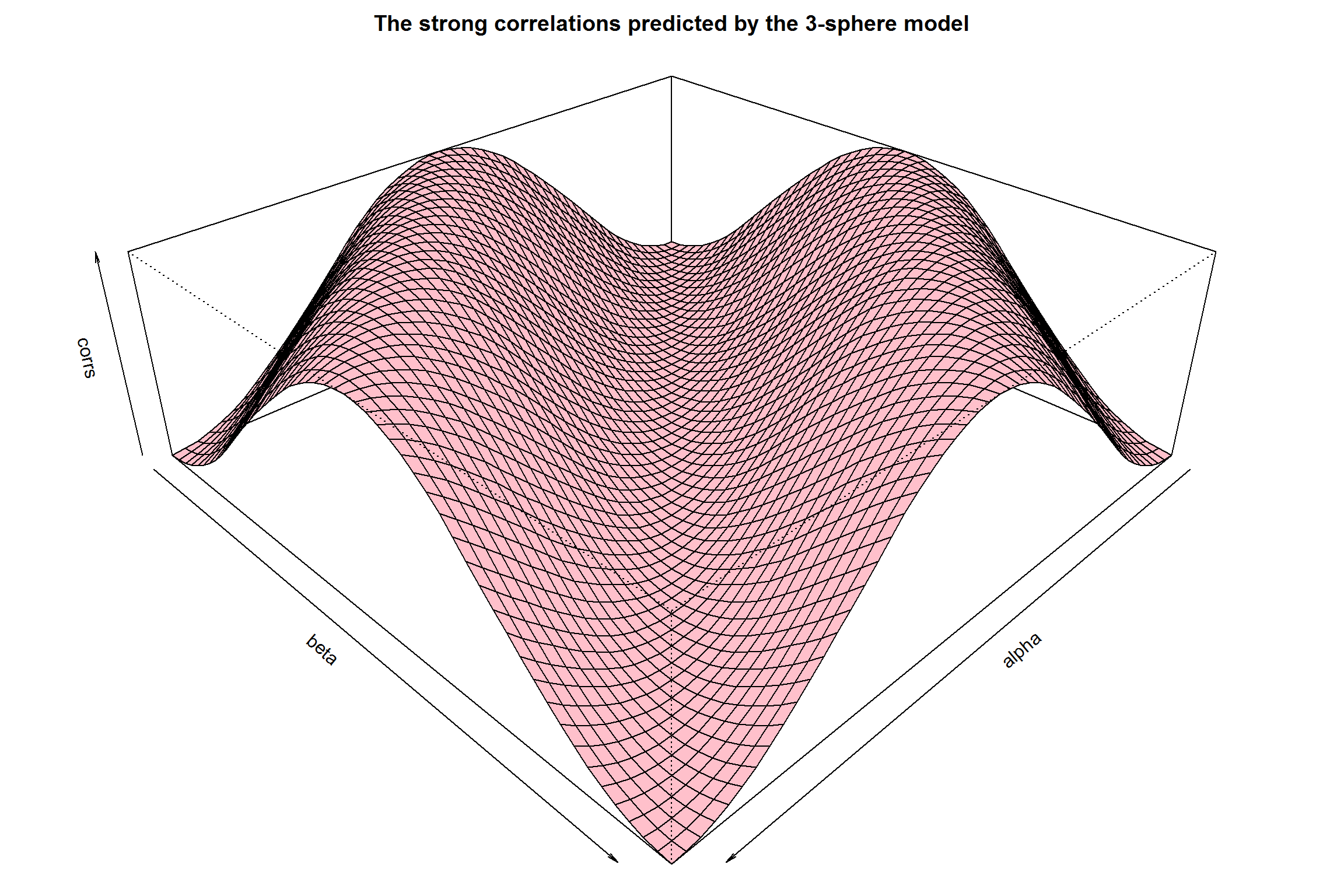# A simulation of the S^3 model for the EPR-B correlation:

by Joy Christian

Einstein Centre for Local-Realistic Physics, 15 Thackley End, Oxford OX2 6LB, United Kingdom

Publication date: 1 June 2015

``````# For a theoretical background, please see http://arxiv.org/abs/1405.2355

# and http://www.sciphysicsforums.com/spfbb1/viewtopic.php?f=6&t=188#p5129``````
``````Angles = seq(from = 0, to = 360, by = 7.2) * 2 * pi/360

K = length(Angles) # The total number of angles between 0 and 2pi

corrs = matrix(nrow = K, ncol = K, data = 0) # Container for correlations

Ns  = matrix(nrow = K, ncol = K, data = 0) # Container for events A and B

Js  = matrix(nrow = K, ncol = K, data = 0) # Container for "zero" events

Ls  = matrix(nrow = K, ncol = K, data = 0) # Container for initial states``````
``````# A canvas of auxiliary pre-ensemble (analogous to pre-reduced phase space):

M = 10^4 # Size of the pre-ensemble. Next one can try 10^5, or even 10^6

r = runif(M, 0, 2*pi) # M uniformly distributed numbers between 0 and 2pi

z = runif(M, -1, +1) # M uniformly distributed numbers between -1 and +1

h = sqrt(1 - z^2)

x = h * cos(r)

y = h * sin(r)

e = rbind(x, y, z)  # A 3xM matrix, with M columns of e-vectors representing
# the x, y, z coordinates of uniformly distributed points on an S^2. In what
# follows these M number of pre-states will define an auxiliary pre-ensemble``````
``````# Defining the metrical and topological structures on S^3:

# So far we have set the variables in R^3. We now construct a metric on S^3

s = runif(M, 0, pi) # Initial states of the spins are the pairs (e, s) within S^3

f = -1 + (2/sqrt(1 + ((3 * s)/pi))) # For details see the paper arXiv:1405.2355

g = function(u,v,s){ifelse(abs(colSums(u*v)) > f, colSums(u*v), 0)}

# g(u,v,s) is non-vanishing only if |u.v| > f(s) ; g(u,v,s) --> "dot" as s --> pi

# Defines an inner product on S^3, thus changing the space from R^3 to S^3

# colSums(u * v) = u.v = the standard inner product between u and v in R^3

# u and v are orthogonal to each other in S^3 when abs(colSums(u * v)) < f

# The metric g(u,v,s) reduces to the standard Euclidean metric in R^3 for f --> 0``````
``````# Computing the "quantum" correlations:

for (i in 1:K) {
# i = 5
alpha = Angles[i]
a = c(cos(alpha), sin(alpha), 0)  # Measurement direction 'a'

for (j in 1:K) {
# j = 23
beta = Angles[j]
b = c(cos(beta), sin(beta), 0)  # Measurement direction 'b'

A = +sign(g(a,e,s))  # Alice's measurement results A(a, e, s) = +/-1

B = -sign(g(b,e,s))  # Bob's measurement results B(b, e, s) = -/+1

N = length((A*B)[A & B]) # Number of all possible events observed in S^3

corrs[i,j] = sum(A*B)/N  # Product moment correlation coefficient E(a, b)

# ----------------------------------------------------------------------

# Metric g(u,v,s) forbids the results (0,+), (0,-), (+,0), (-,0), & (0,0)

# Probabilities for (0,+), (0,-), (+,0), (-,0), and (0,0) vanish exactly

Cuu = length((A*B)[A > 0 & B > 0])   # Coincidence count of (+,+) events

Cdd = length((A*B)[A < 0 & B < 0])   # Coincidence count of (-,-) events

Cud = length((A*B)[A > 0 & B < 0])   # Coincidence count of (+,-) events

Cdu = length((A*B)[A < 0 & B > 0])   # Coincidence count of (-,+) events

# corrs[i,j] = (Cuu + Cdd - Cud - Cdu) / (Cuu + Cdd + Cud + Cdu) # = -a.b

# ----------------------------------------------------------------------

# There are no "0 outcomes" within S^3: Cou = Cod = Cuo = Cdo = Coo = 0

Cou = length((A*B)[g(a,e,s) & A == 0 & B > 0])  # Number of (0,+) events

Cod = length((A*B)[g(a,e,s) & A == 0 & B < 0])  # Number of (0,-) events

Cuo = length((A*B)[A > 0 & B == 0 & g(b,e,s)])  # Number of (+,0) events

Cdo = length((A*B)[A < 0 & B == 0 & g(b,e,s)])  # Number of (-,0) events

Coo = length((A*B)[g(a,e,s) & A == 0 & B == 0]) # Number of (0,0) events

# Coo = length((A*B)[A == 0 & B == 0 & g(b,e,s)]) Number of (0,0) events

CoB = length(A[g(a,e,s) & A == 0])   # Number of A = 0 events within S^3 regardless of B events

CAo = length(B[g(b,e,s) & B == 0])   # Number of B = 0 events within S^3 regardless of A events

# -------------------------------------------------------------------------------

# corrs[i] = sum(A)/length(A)  # Verifies < A > = 0 for all 'a' regardless of 'b'

# corrs[j] = sum(B)/length(B)  # Verifies < B > = 0 for all 'b' regardless of 'a'

# -------------------------------------------------------------------------------

# Computing the same correlations with the standard Euclidean metric in R^3

o = x[A & B]
p = y[A & B]
q = z[A & B]

w = rbind(o,p,q) # N vectors in R^3 representing the initial states within S^3

a.w = colSums(a*w) # Standard inner product in R^3 between the vectors a and w
b.w = colSums(b*w) # Standard inner product in R^3 between the vectors b and w

# corrs[i,j] = sum(-sign(a.w)*sign(b.w))/N # Another way of calculating E(a, b)

# -----------------------------------------------------------------------------

Ns[i,j] = N # The total number of all possible events observed by Alice and Bob

a.e = abs(colSums(a*e))
b.e = abs(colSums(b*e))

L = length(s[a.e > f & b.e > f]) # The number of initial states (e,s) within S^3

Ls[i,j] = L   # The total number of initial states (e,s) emitted from the source

J = length((A*B)[g(a,e,s) & A == 0 & g(b,e,s) & B == 0]) # Number of null events

J = length((A*B)[g(a,e,s) & A == 0 | g(b,e,s) & B == 0]) # Number of null events

Js[i,j] = J # Total number of "vanishing" or "zero" events "observed" within S^3
}
}``````
``(Cou) # This and the following "0" results can be checked for any fixed angles alpha and beta by fixing i and j``
``##  0``
``(Cod)``
``##  0``
``(Cuo)``
``##  0``
``(Cdo)``
``##  0``
``(Coo)``
``##  0``
``(CoB)``
``##  0``
``(CAo)``
``##  0``
``# Thus "0 outcomes" simply do not exist within S^3: Cou = Cod = Cuo = Cdo = Coo = CoB = CAo = 0``
``(W = length(w)/3) # Total number of w vectors``
``##  6686``
``(N) # = length((A*B)[A & B])``
``##  6686``
``(P = length((A*B)[abs(A*B) > 0]))``
``##  6686``
``````# N = P again proves the non-existence of the outcomes (0,+), (0,-), (+,0), and (-,0), whereas
# the (0,0) outcomes are proven to be non-existent in S^3, once defined by the metric g(u,v,s)``````
``````# Nota bene: According to the S^3 model the pre-ensemble M and the pre-states e do not exist in Nature.
# Only S^3, and the states (e, s) within S^3, exist in Nature. Therefore what is physically meaningful
# is the ratio N/L of the total number N of events observed by Alice and Bob and the total number L of
# the complete or initial states (e, s) within S^3. This ratio does not depend on the settings a and b,
# as proved by the graph below: N/L = 1, independently of a and b. Consequently the probability density
# rho(e, s) of the complete or initial states (e, s) also remains independent of the settings a and b.
# In other words, the seeming dependence of rho(e, s) on a and b is with respect to the gauge-dependent
# pre-ensemble M of the pre-states e, not with respect to the true ensemble L of the true states (e, s).``````
``````par(mar = c(0, 0, 2, 0))
persp(x = Angles, y = Angles, main = "Ratio N / L in S^3 = Number N of simultaneous events / Number L of initial states (e, s); from 0 to 1", z = Ns/Ls, zlim = c(0, 1), col = "bisque", theta = 135, phi = 30, scale = FALSE, xlab = "alpha", ylab = "beta", zlab = "N / L")````````````par(mar = c(0, 0, 2, 0))
persp(x = Angles, y = Angles, main = "Ratio J / L in S^3 = Number J of vanishing or zero events / Number L of initial states (e, s); from 0 to 1", z = Js/Ls, zlim = c(0, 1), col = "darkseagreen1", theta = 135, phi = 30, scale = FALSE, xlab = "alpha", ylab = "beta", zlab = "J / L")````````````par(mar = c(0, 0, 2, 0))
persp(x = Angles, y = Angles, main = "The strong correlations predicted by the 3-sphere model", z = corrs, zlim = c(-1, 1), col = "pink", theta = 135, phi = 30, scale = FALSE, xlab = "alpha", ylab = "beta")````````````par(mar = c(0, 0, 2, 0))
QM = matrix(nrow = K, ncol = K, data = sapply(Angles, function(t) -cos(t - Angles)), byrow = TRUE)
persp(x = Angles, y = Angles, main = "The corresponding quantum mechanical correlations", z = QM, zlim = c(-1, 1), col = "khaki", theta = 135, phi = 30, scale = FALSE, xlab = "alpha", ylab = "beta")``````